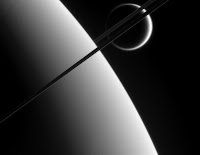Page Views on Nuit Blanche since July 2010

My papers on ArXiv:
Approximating Kernels at the speed of Light
&
Imaging with Nature

LightOn
LinkedIn (727)|| on CrunchBase || our Blog
(2452)
(3967)
(1333)||
Attendant references pages:
The Advanced Matrix Factorization Jungle Page ||

Paris Machine Learning
@Meetup.com (8016 members) || @Archives

Tuesday, January 15, 2008

Monday Morning Algorithm 9: Implementation of Sparse Measurement Matrices for Compressive SamplingOk, so it's not Monday, at least I thought about the algorithm on Monday Morning so there. Last week I mentioned the work of Radu Berinde and Piotr Indyk on Sparse Recovery Using Sparse Random Matrices ( an older version of this paper is here). Since this is a potentially ground breaking work with regards to the hardware implementation of Compressive Sampling, I decided to implement a non-optimized version of the measurement matrix construction of the paper. As usual if you find a mistake please let me know. Also all the codes produced in the Monday Morning Algorithm Series can be found here.

clear
% Algorithm implementing the Sparse Measurement Matrix
% construction of Radu Berinde and Piotr Indyk in
% "Sparse Recovery Using Sparse Random Matrices"
% d is the number of ones per column
% m is the number of rows
% n is the number of columns
% generally m should be much less than n
% n is limited by (m,d) or
% factorial(m)/(factorial(d)*factorial(m-d))
% Written by Igor Carron
d=3
% m rows
m=5;
% n columns
n=10;
% with these parameters it works with n=10
% but it does not work with n greater than 10
A=zeros(m,n);
jj = 0;
for i=1:n
while sum(A(:,i),1)~=d | jj==47
jj=0;
A(:,i)=zeros(m,1);
for j=1:d
A(round((m-1)*rand(1,1))+1,i)=1;
end
v1 = diag(A(:,i))'*ones(m,n);
w = A - v1;
for j=1:i-1
if w(:,j)== zeros(m,1)
jj = 47;
end
end
end
end
% Measurement Matrix is A

Some people might think there is an error in j = 47, they think it is j =42. They are wrong. Also while this is a binary matrix, it can be used with non-binary objects. [ Update: I have made it a function and it is available here. It is working as nicely as the normal measurement matrices on the example set I used it on].

Credit Photo: NASA/JPL/ Space Science Institute, Saturn and Titan on June 9, 2006.